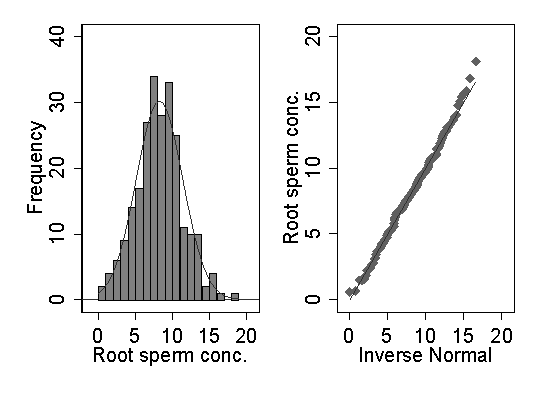# Solution to question on the distribution of sperm count, 5

We cannot use the exact probabilities or mean and variance of the Poisson distribution, because the original counts have been multiplied by an unknown constant. We can use the fact that a square root transformation is the transformation which will make a Poisson distribution Normal. If the square root is Normal, the observations may have a distribution related to the Poisson. Below are a histogram and Normal plot for the square root of the sperm concentrations. The fit to the Normal is much better than for either the untransformed or log-transformed data. We can conclude that there is good evidence that a Poisson distribution may underlie the distribution of sperm concentration.d